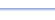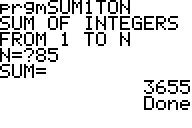Program: SUM1TON

SUM1TON

• Finds the sum of the integers from 1 to n.

Useful for:

• SAT
• ACT
• Algebra 1
• Algebra 2
• Precalculus
• Calculus

See full list of programs

Example

Q: Find the sum of the integers from 1 to 85, inclusive.

Solution:

Run SUM1TON.  How?

Enter N=85

The program reports:

Interpretation:
The sum is 3655.